Contents

2.1. Refal Graphs

I will reveal a small secret. Our supercompilers do not actually use Refal as the language of object programs; they use the language of pattern-matching graphs, also referred to as Refal graphs. The program in Refal to be supercompiled is first automatically translated into the graph form, and the output of the SCP is also a Refal graph.

Algebraically, a pattern-matching graph is a sum of products of three varieties of the operation of pattern-matching, with a product implying sequential, and a sum parallel, execution. A contraction is a pattern matching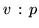, where v is a variable and p is a rigid pattern; we shall denote this contraction as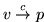. An assignment is a pattern matching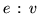, where e is an expression and v a variable; we shall denote this assignment as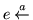v. A restriction is (# G), where G is a contraction graph, i.e., a pattern-matching graph which consists only of contractions. It is evaluated either to Z(impossible operation, failure), if at least one contraction path in G is successful, or to the identity operation I (do nothing) otherwise.

This notation may seem unusual (especially that of an assignment), but it is logical and quite convenient. It is derived from the following two principles. (1) On the left side we have bound (old, defined) variables; on the right side free (new, to be defined) variables. (2) When the operation is understood as a substitution, the arrow is directed from the variable to its replacement.

Examples. x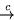s.1 x'a'  is a contraction for x. If the value of x is 'koshka', after the execution of this contraction x becomes 'oshk', and a new variable s.1 becomes defined and has the value 'k'. If x is 'kot' the result is the impossible operation Z (failure of matching). Further, this contraction can be decomposed into a product of elementary contractions: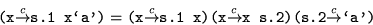An example with a restriction: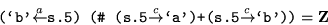I have developed a set of relations of equivalency for the algebra of pattern-matching operations, and the programming of SCP-3 was based on it, but, unfortunately, this theory is not yet published; the initial stages of the theory can be found in . The most important equivalence is a clash between an assignment and a contraction for the same variable, which is resolved in a matching: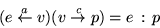Thus the example above can be seen as the equation:Here is an example of resolution which includes the contraction of a bound variable:

('kot's.2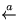x)(xx'a')=(s.2'a')('kot'x)

To give an example of a function definition in the graph form, here is the graph for the iterative program of changing each 'a' to 'b', where nodes [n] correspond to configurations of the Refal machine:

  ([]y)   (xs.1 x) { (s.1'a') (y'b'y)  +(# s.1'a') (y s.1y)  } +(x[]) []out

With Refal graphs, driving is an application of commutation relations for pattern-matching operations. A walk in the normal form, in which it appears in function definitions and represents one step of the Refal machine, has the structure CRA, where the letters stand for Contraction, Restriction and Assignment, respectively. A walk representing two steps is C1R1A1C2R2A2. Resolving the clash A1C2 and then adjusting contractions and restrictions according to commutation relations, we return the walk to the normal form CRA: we have made one step of driving.

Contents  Next